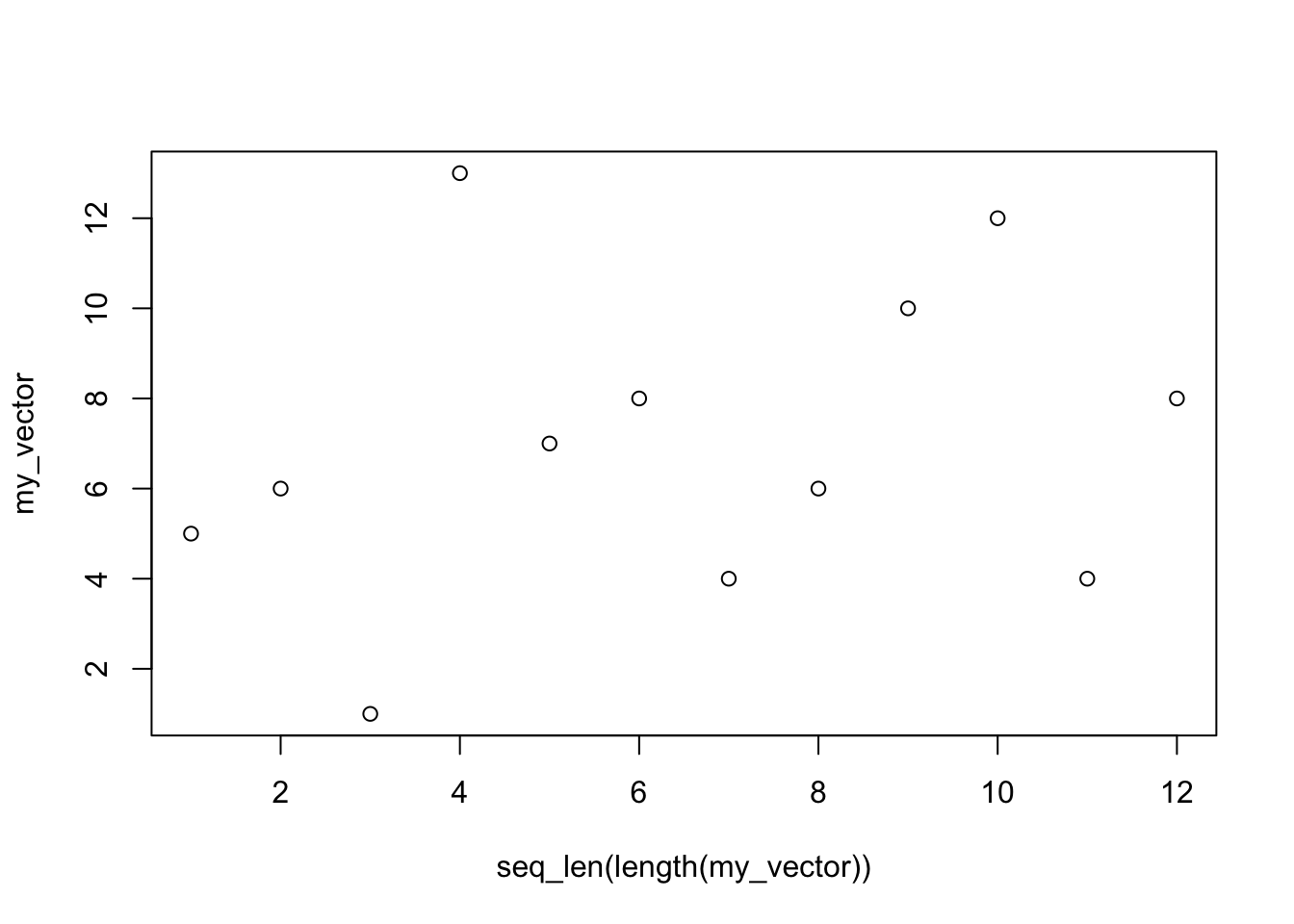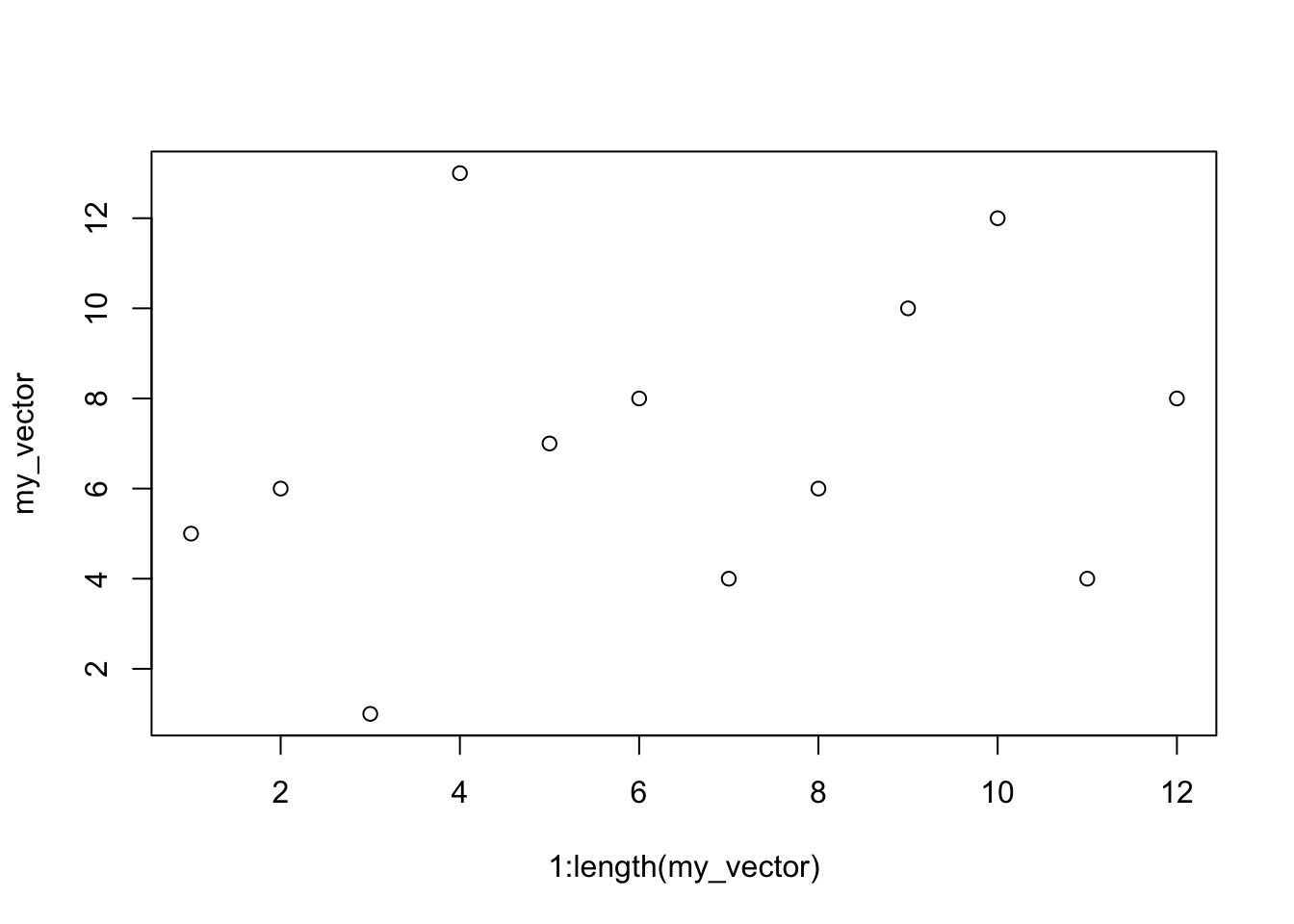# 1 Goal

The goal of this tutorial is to understand the use of the seq_len function. In addition we will see different ways to declare sequences in R.

# 2 seq_len: How and why

The seq_len(number) function creates a sequence that starts at 1 and with steps of 1 finishes at the number value. A common use of this function is to create indexes that match the length of a vector in order to make plots.

## 2.1 seq_len use example

``````# We create a sequence that starts at 1 and ends at 50
seq_len(50)``````
``````##    1  2  3  4  5  6  7  8  9 10 11 12 13 14 15 16 17 18 19 20 21 22 23
##  24 25 26 27 28 29 30 31 32 33 34 35 36 37 38 39 40 41 42 43 44 45 46
##  47 48 49 50``````
``````# Let's create a vector of length 12
my_vector <- c(5, 6, 1, 13, 7, 8, 4, 6, 10, 12, 4, 8)
length(my_vector)``````
``##  12``
``````# We can use the length of the vector to create an index
seq_len(length(my_vector))``````
``##    1  2  3  4  5  6  7  8  9 10 11 12``
``````# Now we can use the seq_len function to plot this vector in two dimensions using this index
plot(seq_len(length(my_vector)), my_vector)``````There are other ways to create sequences that we are going to show in this tutorial. However the seq_len function is often used because it only takes one parameter and it is very easy to identify in a dense and complex code.

## 2.2 Summary

At this point the use of seq_len is very clear. It is a function of one parameter that creates a sequence from 1 to this parameter using steps of 1. It’s easy to spot in the code and it’s commonly used to create indexes using a vector. Now feel free to use this function or follow the tutorial to learn different alternatives.

# 3 Alternative function

``````# We can in R easily create sequences with steps of 1 using the : command
1:12``````
``##    1  2  3  4  5  6  7  8  9 10 11 12``
``10:20``
``##   10 11 12 13 14 15 16 17 18 19 20``
``````# We can repeat the previous example using this command
plot(1:length(my_vector), my_vector)``````# 4 The seq main function

``````# Now it's a good time to read the help page of the seq_len function
# ?seq_len

# We see that it descends from a main function called seq

# This function is more general and can take many parameters that make it very useful
# Let's start creating a sequence from 1 to 20
seq(1, 20)``````
``##    1  2  3  4  5  6  7  8  9 10 11 12 13 14 15 16 17 18 19 20``
``````# We can also use this function in the main example of this tutorial
# However this function takes two parameters and it's not very practical
plot(seq(1,length(my_vector)), my_vector)``````